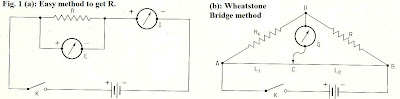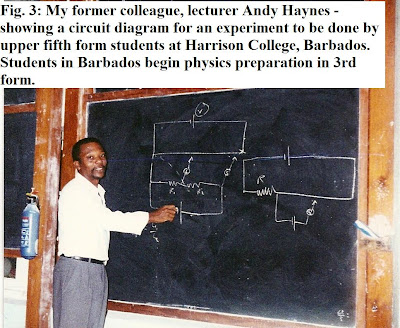## Sunday, June 26, 2011

### Introduction to Basic Physics (Electric Circuits) Pt. 21In this instalment we begin the examination and investigation of simple electric circuits, starting with some basic rules and principles and very simple experiments to determine the resistance, R, in a circuit. There are two methods that can be used one I call "simple", otherwise known as the ammeter-voltmeter method, and the other which is more complex (shown in Fig. 1(b). The latter actual practical set up is also shown in Fig. 2.

Generally, at Harrison College, we employed the simple A-V method for the introductory physics students in the 3rd form (equivalent to the U.S. 9th grade) and used the Wheatstone Bridge method for the Upper fifth form (equivalent to the U.S. 12th grader, or HS senior). Thus, by the time the Barbadian physics student arrives at his senior year he's already been well exposed to simple electric circuits, and knows how to set up both series and parallel circuits (which we will see in the next instalment).

Some Basics:

1.Ohm's Law:

In effect, this is what the student is really seeking to show in the experiment:

I = V/R

where I is the current (in amperes) and V the voltage in volts, while R is the resistance in ohms. In terms of the units then:

Amperes (or 'amps') = Volts/ Ohms

2. Internal Resistance

Technically speaking, every battery, or source of emf (electro-motive force) also has an internal resistance, r. Thus, the theoretical emf (E-t) will always be larger than the actual, measured emf,E. And hence also, any terminal voltage in the circuit (call it V(ab)) will be less than E. Thus:

V(ab)= E - Ir

or, if an additional resistance R is connected:

E - Ir = IR, or

I = E / (R + r)

The fine points of (2) are usually not introduced until the upper fifth form.

3. Resistivity

This features prominently when lengths of resistance wire come into play and hence the resistance will change depending on the length. If rho denotes resistivity of a material, e.g. metal wire, and the wire is L feet long, then the resistance R (in ohms) is:

R = rho (L)/ A

where A is the cross-sectional area of the wire.

Again, (3) is a consideration usually left until the more advanced levels.

I. Ohm's Law by the Simple Method

The student is issued an ammeter (with letter I adjacent, for current) to measure the amperes, and a voltmeter (with E adjacent) to denote volts or emf at that point, and a set of 5 unknown or test resistors, as well as a battery or other emf source.

For each test resistor inserted, the student records the voltage and current in volts and amps, then obtains the resistance using:

R = E/ I = V/I

At the end of the experiment the student is given the actual resistor values which he must compare with the empirical or test values he found, and then estimate the percentage error for each.

II. Wheatstone Bridge Method:

This circuit is connected as shown in diagram Fig. 1(b). In this case, the unknown resistance R(x) is wired in as shown. The instrument denoted by (G) is a galvanometer. The known resistance is denoted R, and the student adjusts or moves a clip along a length of wire from A to B yielding different lengths L1 and L2. At specific points where 'C' is located on AB, the galvanometer will read 0 (N.B. the value R should be chosen before hand so that point C falls on the middle third of AB when G reads zero).

Practical example:

In performing the Ohm's law experiment (to find an unknown resistance R(x)) a student at Harrison College makes the following measurements:

Length AC = 35 cm

Length CB = 65 cm

R = 5 ohms

G = 0

Using this data, find the value of R(x):

Solution:

R(x) / R = (AC)/ (BC)

R(x)/ 5 ohms = 35/65 = 7/13

Therefore: R(x) = 5 ohms (7/13) = 2.7 ohms

Problems:

1) A 12 V battery has an internal resistance of 2 ohms. If it is connected in series with a voltmeter and another resistance R = 4 ohms, what would the voltmeter read? What would an ammeter read placed in the same circuit?

2) A Wheatstone Bridge circuit is connected as shown in Fig. 1(b). The galvanometer is found to read zero when point C is located exactly midway along a wire 1m in length (e.g. connecting A and B in the diagram). A known resistance coil R is used which is made of copper (rho = 1.72 x 10^-8 ohm-m) and is 50 m long, wound tightly in a coil.

a) If the cross sectional area A = πr^2 and r = 0.001m, find the value of R(x).

b) If a new resistor R made up of 100m length of the same copper wire is then used, then how must the lengths L1 and L2 change to achieve a galvanometer reading of zero?